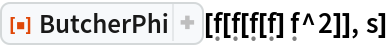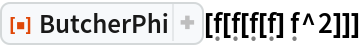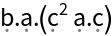Function Repository Resource:

# ButcherPhi

Compute the weight of a Butcher tree

Contributed by: Wolfram Research
 ResourceFunction["ButcherPhi"][tree,s] gives the weight of tree in an s-stage Runge-Kutta method. ResourceFunction["ButcherPhi"][tree] gives the weight of tree in stage-independent tensor notation.

## Details and Options

The weight of tree is the left-hand side of the order conditions imposed by tree.
The following option can be given:
 "RungeKuttaMethod" "Implicit" type of Runge-Kutta method
Valid settings for "RungeKuttaMethod" are "DiagonallyImplicit", "Explicit" and "Implicit".

## Examples

### Basic Examples (2)

Compute the elementary weight function imposed by f[f[f[f]f^2]] for an s‐stage method:

 In:=Out=Repeat, giving the result in stage-independent tensor notation:

 In:=Out=## Version History

• 1.0.0 – 08 November 2019